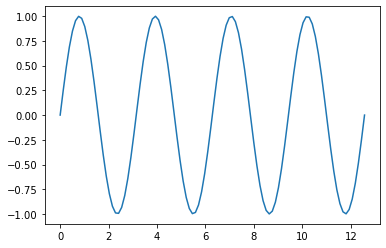# Content in Jupyter notebooks

You can also have content as Jupyter notebooks. This is one of them! Notebooks aren’t executed so they will only contain output if you’ve run the notebook yourself.

## Import packages

The Scipy stack

``````import numpy as np
import matplotlib.pyplot as plt
``````

## Make a plot

Generate a figure.

``````time = np.linspace(0, 4 * np.pi, 100)
data = np.sin(2 * time)
``````
``````plt.figure()
plt.plot(time, data)
``````
``````[<matplotlib.lines.Line2D at 0x7f81fae0b280>]
``````